A very basic problem in computer network programming: how to represent the data interacted between client and server, think about this problem before reading on.

## Consensus and Protocols

The problem is not as simple as it seems, because the client process and the server process are running on different machines, which may run on different processor platforms, may run on different operating systems, may be written in different programming languages, how does the server recognize what data the client is sending?

Something like this.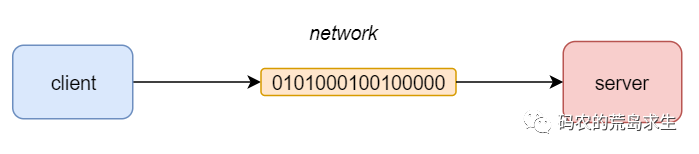The client sends a piece of data to the server.

 ``````1 `````` ``````0101000100100001 ``````

How can the server know how to “interpret” this data?

Obviously, the client and server must first reach some kind of consensus on how to interpret the data before sending it, which is called a protocol.

The protocol here could be something like this: “interpret every 8 bits as an unsigned number”, if the protocol is like this, then the server receives the binary string and parses it as 81(01010001) and 33(00100001).

Of course, the protocol here could also be something like “interpret every 8 bits as an ASCII character”, then the server receives the binary string and parses it as “Q!

As you can see, the same binary string can be interpreted completely differently in different “contexts/protocols”. This is the fundamental reason why computers can handle very complex tasks even though they only know 0 and 1, because everything can be coded as 0 and 1, and we can also parse the information we want from 0 and 1, which is called codec technology.

In fact, not only 0 and 1, we can also encode information as Morse code, etc., but computers are just good at handling 0 and 1.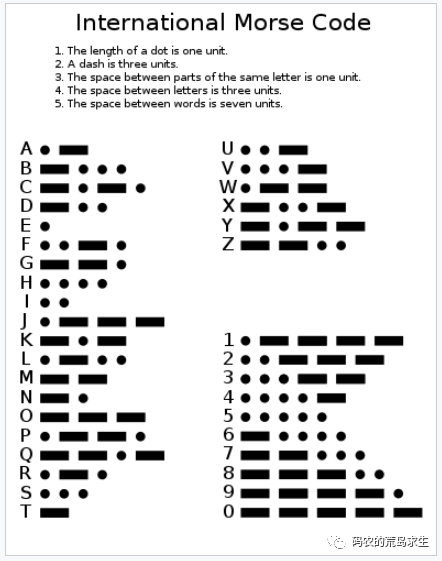## Remote Procedure Call: RPC

As programmers, we know that the client and the server will not simply pass a string of numbers and characters between them, especially in the scenario of back-end services of large Internet companies.

When we search for products in e-commerce apps, call cabs in taxi apps and watch short videos, behind each request there are a lot of interactions between services in the backend, like this.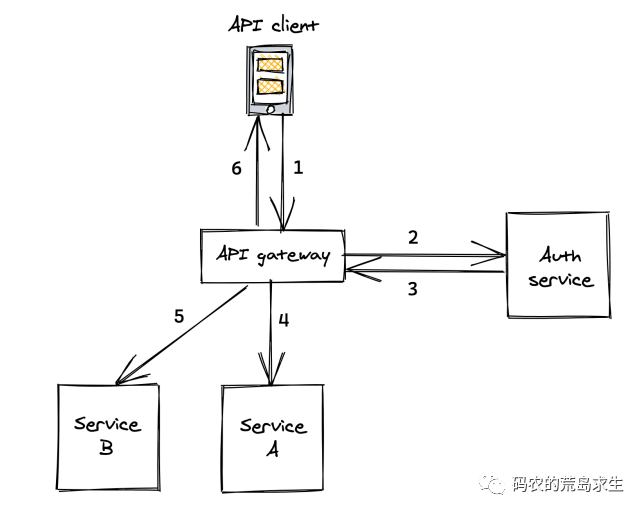Complete a client request gateway this service to call more than N downstream services, the so-called call is to say that the A service to the B service to send a piece of data (request), the B service received this data after the implementation of the corresponding function, and the results will be returned to the A service.

Only for service A does not want to care about the underlying details of the network transmission, if you can call remote services like a local function is good, this is the so-called RPC, the classic implementation is as follows.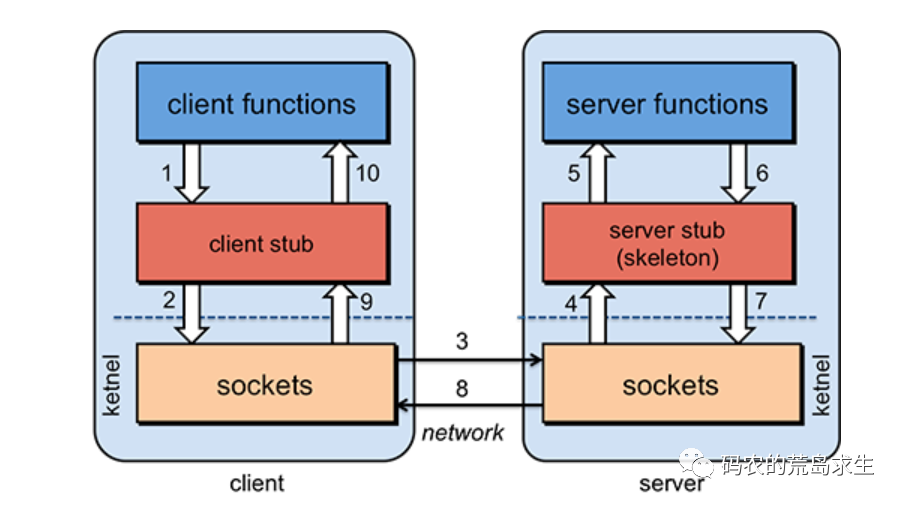RPC to the upper layer to provide the same interface as ordinary functions, but in the implementation of the encapsulation of the underlying complex network communication, RPC framework is one of the cornerstones of the current Internet back-end. Many so-called Internet back-end positions are nothing more than a heap of business logic on top of this foundation.

In this paper we do not care about the details, here we only care about how the client is encoded in the network layer request parameters, how the server decodes the request parameters, which is the question raised at the beginning of this paper.

## coding and decoding of information

Before thinking about how to codec we must be aware that

• client and server may be written in different languages, your codec scheme must be common and not language bound
• Performance issues of codec methods, especially for time-critical services

First of all, the most important thing we should be able to think of is to represent it as plain text.

Plain text has never been a very friendly information carrier, why? Simply, because humans (us) can read it directly, as in this paragraph.

 `````` 1 2 3 4 5 6 7 8 9 10 11 12 13 14 15 16 `````` ``````{ "widget": { "window": { "title": "Sample Konfabulator Widget", "name": "main_window", "width": 500, "height": 500 }, "image": { "src": "Images/Sun.png", "name": "sun1", "hOffset": 250, "vOffset": 250, }, } } ``````

Is it clear, at a glance, as long as we realize the agreed text structure (that is, syntax), then the client and server can use this text to encode as well as decode information, regardless of whether the client and server are running on x86 or Arm, 32-bit or 64-bit, running on Linux or windows, or large end or small end, they can communicate without any obstacles.

So here, the syntax of text is a protocol.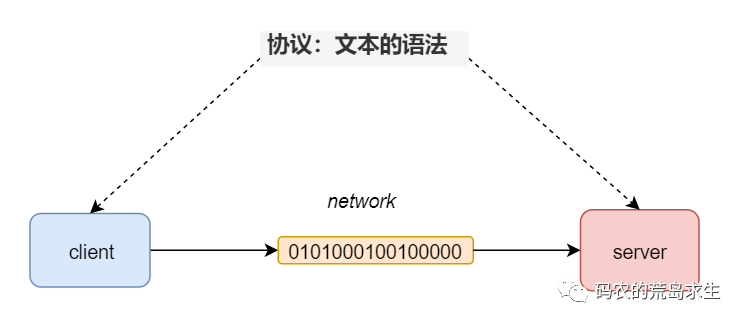By the way, you have specified the syntax of the text, in fact, is equivalent to the invention of a language .

The language used here as an example is the so-called Json, except that the language json is not used to represent logic (code) but to store data.

Json was proposed by this old man.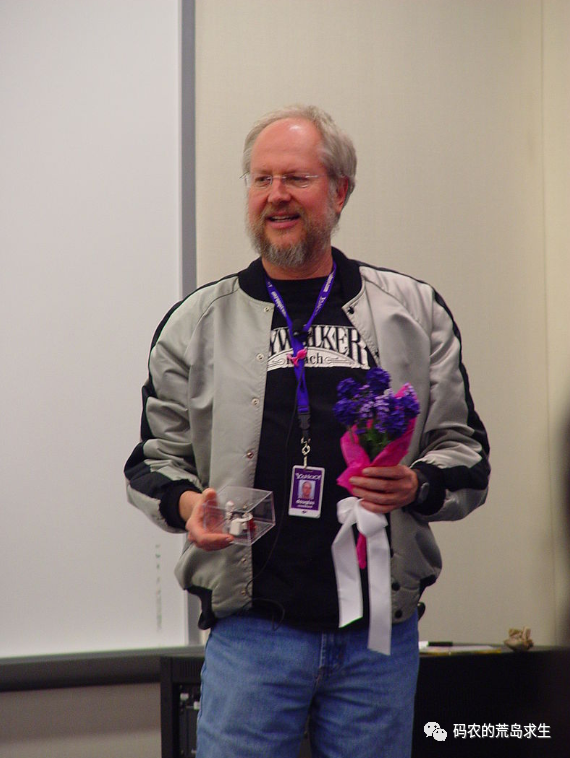In addition to Json, another method of representing data stored in text is XML.

 ``````1 2 3 4 5 6 `````` `````` Tove Jani Reminder Don't forget me this weekend! ``````

It is not so easy to read compared to Json, which has gradually replaced XML in the web domain since its appearance.

Json can work very well when the amount of data on both ends is small - like the interaction between the browser and the server.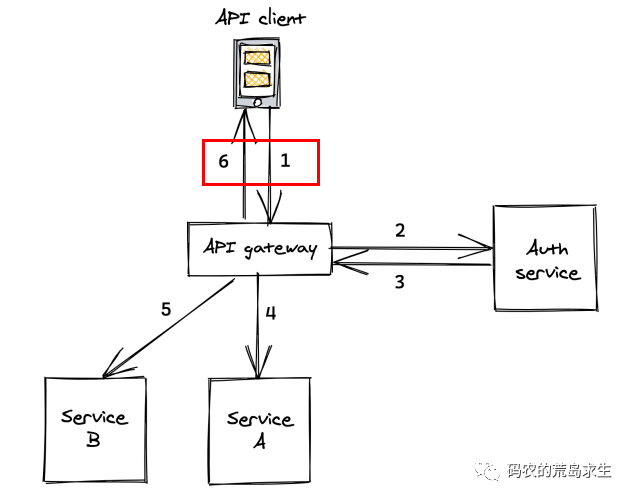However, it is not the same for the interaction between back-end services. RPC calls between back-end services may transfer a large amount of data, and if all data is represented in plain text form, then both network bandwidth and performance may be poor.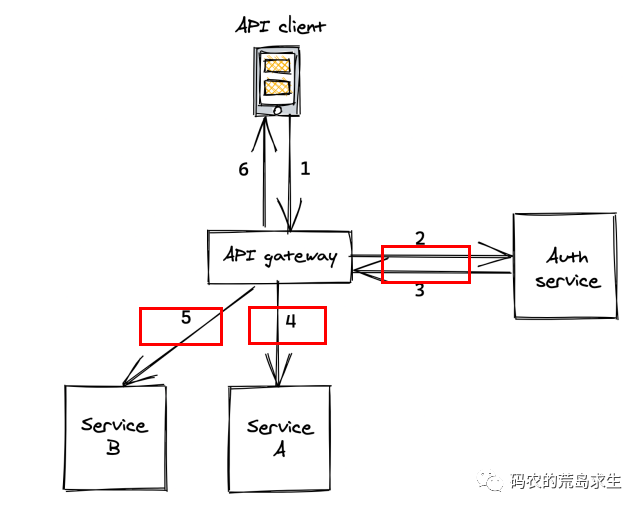In this scenario, Json is not the best option, one of the main reasons lies in the performance and the size of the data.

We know that textual representation is the most human friendly, not so for machines, which are still best for 01 binary.

So is there a binary encoding method? The answer is yes, this is the current Internet backend in the popular protobuf, Google Inc. open source project.

So what is the magic of protobuf?

Suppose the client side wants to transmit a message to the server side: “I have an id with the value 43”, which is represented in XML as follows.

 ``````1 `````` ``````43 ``````

count how many bytes this piece of data takes up, and it is clear that it is 11 bytes.

And what if we use json to represent it?

 ``````1 `````` ``````{"id":43} ``````

count how many bytes this data occupies, which is clearly 9 bytes.

And what if we use protobuf to represent it? It goes like this.

 ``````1 2 3 4 5 6 7 8 `````` ``````// Message Definition message Msg { optional int32 id = 1; } // Instantiation Msg msg; msg.set_id(43); ``````

Where the definition of Msg looks more complex than Json and XML, but these are only meant to be read by humans, and these will be further processed by protbuf and eventually encoded as follows.

 ``````1 `````` ``````082b ``````

That is, 0x08 and 0x2b, how many bytes does this take up? The answer is 2 bytes.

From 9 bytes in json to 2 bytes in protobuf, the data size is reduced by more than 4 times. The reduction in data size means.

• Less network bandwidth
• Faster parsing speed

So how does protobuf do this?

## How is protobuf implemented?

First, let’s think about the simplest case, how to represent a number.

You may think this is simple. A uniform fixed length, say 64 bits (8 bytes), would work, but the problem is that no matter how small a number is, let’s say 2, then representing 2 in this way would also take up 64 bits (8 bytes).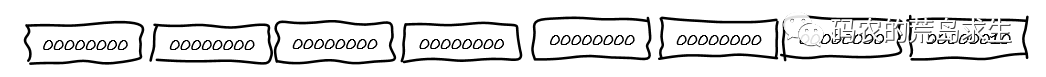It’s too extravagant and wasteful to use 8 bytes when it’s clear that only one byte is needed, and the first ones are all zeros.

Obviously, here we can not use a fixed length to represent the number, but need to use the variable length method to represent.

What do you mean by variable length? It means that if the number itself is large, then it can use more bits, but if the number is small then it should use less bits to represent it, this is called variable length, random and not rigid.

So how does it get longer?

We specify that for each byte, if the first bit is a 1 then it means that the next bit is still to be interpreted as a number, and if the first bit is a 0 then it means that the next byte is not to be used to represent that number.

This means that for each 8 bits (1 byte), it has a payload of 7 bits, and the first bit is only used to mark whether the next byte should still be interpreted as a number.

According to this provision assumes comes such a string of 01 binary.

 ``````1 `````` ``````1010110000000010 ``````

According to the regulations, we first take out the first byte, i.e.

 ``````1 `````` ``````10101100 ``````

At this point we find that the first bit is a 1, so we know that the next byte also belongs to that number, and removing the 1 from the current byte is.

 ``````1 `````` ``````0101100 ``````

Then we look at the next byte.

 ``````1 `````` ``````00000010 ``````

We find that the first bit is 0, so we know that the next byte does not belong to the number anymore.

Next we flip the parsed 0101100 (first byte minus the first bit) and the second byte 0000010 (second byte minus the first bit) and splice them together. The reason for the flip here is that we specify that the high bit of the number comes after.

The process is as follows.

 ``````1 2 3 4 5 6 7 8 9 `````` `````` 1010110000000010 -> 10101100 | 00000010 // Parsing yields two bytes _ _ -> 0101100 | 0000010 // Each remove the highest bit -> 0000010 | 0101100 // Two byte flipping order 0000010 + 0101100 -> 100101100 // concat ``````

Finally we get 100101100, a binary string representing the number 300.

This variable length representation of numbers is called varint in protobuf.

So with this representation, if the number is larger then more bits are used, if the number is smaller then less bits are used, smart right.

Some of you may have questions here, the method just explained can only represent unsigned numbers, so how do you represent signed numbers? For example, how to represent -2?

### Representation of signed numbers

Following the idea of variable-length encoding just now, -2147483646 should use fewer bits than -2.

However, we know that in the computer world negative numbers are represented using complement, which means that the highest bit (the leftmost bit) must be 1. Suppose we use 64 bits to represent the number, then if we still use complement to represent the number then the negative number will take up 10 bytes of space no matter how big or small it is.

Why 10 bytes?

Don’t forget that the payload of each byte of varint is 7 bits, so for a number that needs a 64-bit representation it needs to be 64/7 rounded upwards which is 10 bytes to represent.

This obviously does not satisfy our requirement for variable length storage of numbers.

How to solve this problem?

Since unsigned numbers can be conveniently encoded in variable lengths, wouldn’t it be better to map signed numbers as unsigned numbers , which is called ZigZag encoding, isn’t that clever, like this.

 `````` 1 2 3 4 5 6 7 8 9 10 11 12 13 `````` ``````Original information After encoding 0 0 -1 1 1 2 -2 3 2 4 -3 5 3 6 ... ... 2147483647 4294967294 -2147483648 4294967295 ``````

This allows us to convert the signed numbers to unsigned numbers, and the receiver receives that data and then recovers the signed numbers.

Now the problem of numbers is completely solved, but this is only the first step of a long journey.

### Field Name and Field Type

For any useful information contains several parts such as

• Field name
• Field type
• Field value

Just as in C/C++ when defining variables.

 ``````1 `````` ``````int i = 100; ``````

Here, the field name is i, the field type is int, and the field value is 100.

We just solved the problem of field value representation with varint and ZigZag encoding, so how to represent the field name and field type?

First of all, for field types is still relatively simple, because the number of field types is not much, protobuf in the definition of six field types.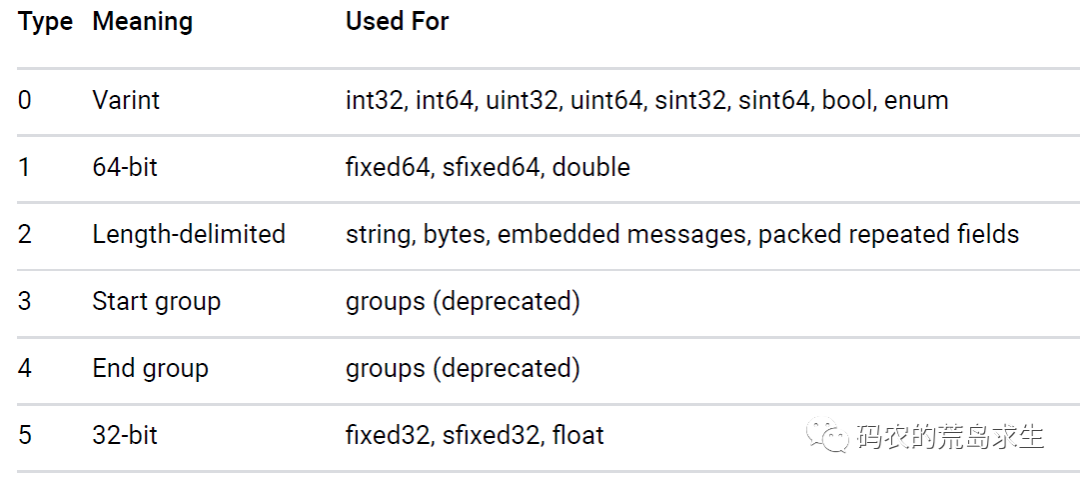For the 6 field types we use 3 bits to represent them and that’s enough.

The next more interesting question is how to represent the field name? Suppose we need to pass a field such as

 ``````1 `````` ``````int long_long_name = 100; ``````

So do we really need to pass so many characters as “long_long_name” to the other side over the network?

Since both sides need to agree, the field “long_long_name” is actually known to both client and server, the only thing they don’t know is “which values belong to which fields”.

To solve this problem, we number each field, for example, if both communicating parties know that the field “long_long_name” has the number 2, then for the following code.

 ``````1 `````` ``````int long_long_name = 100; ``````

This information we only need to pass.

• Field name: 2 (2 corresponds to the field “long_long_name”)
• Field type: 0 (0 means varint type, see above)
• Field value: 100

So we can see that no matter how complex the field name is, it doesn’t affect the space occupied after encoding, the field name doesn’t appear in the encoded information at all, so clever.

### From a macro perspective

We’ve seen how numbers are represented in protobuf as well as field names and field types, now it’s time to look at how multiple fields should be encoded from a macro perspective.

Essentially, a protobuf is encoded to form a series of key-values, each of which corresponds to a field in the proto.

Also known as key-value pairs.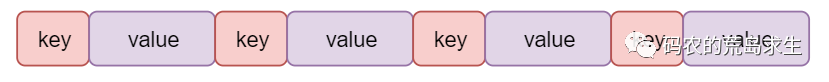where value is relatively simple, that is, the field value; and the field name and field type will be spliced into key. There are 6 types in protobuf, so only 3 bits are needed; the field name only needs to store the corresponding number, and it can be encoded as follows.

 ``````1 `````` ``````(Field Number << 3) | Field Type ``````

Suppose the server receives a key of 0x08, the binary representation of which is as follows.

 ``````1 `````` ``````0000 1000 ``````

Since the key is also encoded using varint, the first bit needs to be removed, so that I get the following.

 ``````1 `````` ``````000 1000 ``````

Depending on how the key is encoded, the next three bits indicate the field type, i.e.

 ``````1 `````` ``````000 ``````

That is, 0, so that we know that the type of the key is Varint (type 0) and the field number is the value of the last 3 bits of the erased, i.e.

 ``````1 `````` ``````0001 ``````

In this way, we know that the key corresponds to a field number of 1, and getting the number we can find the corresponding numbered name according to the number.

### Nested data

Similar to Json and XML, nested messages are supported in protobuf, as follows.

 ``````1 2 3 4 5 6 `````` ``````message SubMsg { optional int32 id = 1; } message Msg { optional SubMsg msg = 1; } ``````

The implementation is also relatively simple, which still follows being encoded to form a series of key-values, except that for nested types of keys, the value is composed of the key-value of the sub-message.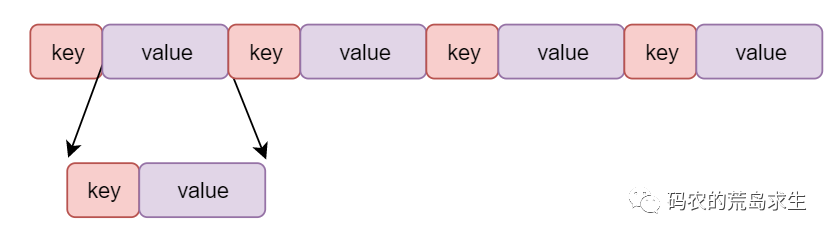### protobuf and compiled languages

Like Json, protobuf is a language that combines the readability of text with the efficiency of binary.

What makes protobuf do this is like C and machine instructions.

C is for programmers and is readable, while machine instructions are for hardware and perform well. The compiler converts C programs into machine-executable machine instructions.

And so is protobuf, which is also a language that encodes well-readable messages into binary so they can be propagated across the network, and the other end can decode them back.

Here the messages defined in protobuf are like C, and the encoded binary messages are like machine instructions.

And protobuf as a de facto language necessarily has its own syntax, which is then as follows.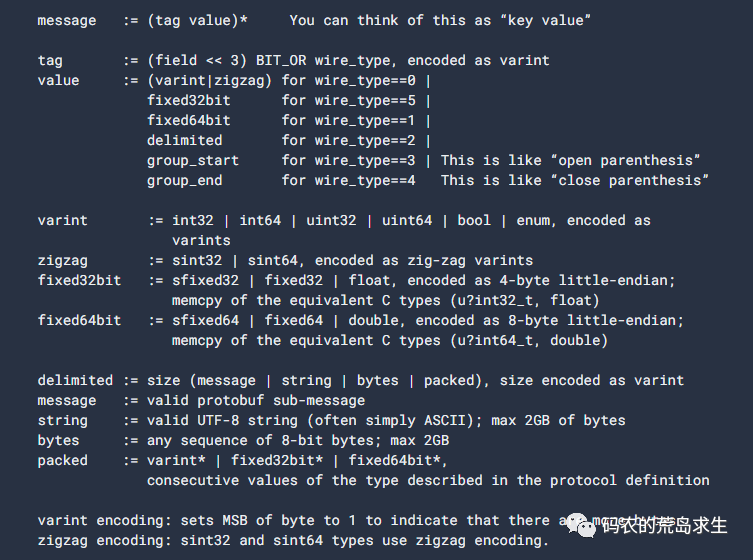How about it, still think the compilation principle is not useful?

It is impossible to invent a technology like protobuf without understanding the compilation principle.

How about it, still think the compilation principle is not useful?

It is impossible to invent a technology like protobuf without understanding the compilation principle.

## Conclusion

As I write this article, I keep lamenting how much time Google’s technology has saved programmers, but at the same time we can see that this cornerstone technology relies on an underlying principle that is very old.

• the coding and decoding of information
• The principle of compilation

How about these are far less interesting than the various popular technologies in the IT world, but it is this simple technology that supports the industry, and now you should be able to understand the importance of the underlying technology.